# Search by Topic

#### Resources tagged with Multiplication & division similar to Magic Crosses:

Filter by: Content type:
Age range:
Challenge level:

### There are 135 results

Broad Topics > Calculations and Numerical Methods > Multiplication & division### Like Powers

##### Age 11 to 14 Challenge Level:

Investigate $1^n + 19^n + 20^n + 51^n + 57^n + 80^n + 82^n$ and $2^n + 12^n + 31^n + 40^n + 69^n + 71^n + 85^n$ for different values of n.### Please Explain

##### Age 11 to 14 Challenge Level:

Visitors to Earth from the distant planet of Zub-Zorna were amazed when they found out that when the digits in this multiplication were reversed, the answer was the same! Find a way to explain. . . .### Slippy Numbers

##### Age 11 to 14 Challenge Level:

The number 10112359550561797752808988764044943820224719 is called a 'slippy number' because, when the last digit 9 is moved to the front, the new number produced is the slippy number multiplied by 9.### X Marks the Spot

##### Age 11 to 14 Challenge Level:

When the number x 1 x x x is multiplied by 417 this gives the answer 9 x x x 0 5 7. Find the missing digits, each of which is represented by an "x" .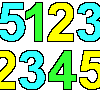### Sept03 Sept03 Sept03

##### Age 7 to 11 Challenge Level:

This number has 903 digits. What is the sum of all 903 digits?### What Two ...?

##### Age 7 to 11 Short Challenge Level:

56 406 is the product of two consecutive numbers. What are these two numbers?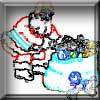### Clever Santa

##### Age 7 to 11 Challenge Level:

All the girls would like a puzzle each for Christmas and all the boys would like a book each. Solve the riddle to find out how many puzzles and books Santa left.### As Easy as 1,2,3

##### Age 11 to 14 Challenge Level:

When I type a sequence of letters my calculator gives the product of all the numbers in the corresponding memories. What numbers should I store so that when I type 'ONE' it returns 1, and when I type. . . .### Multiply the Addition Square

##### Age 11 to 14 Challenge Level:

If you take a three by three square on a 1-10 addition square and multiply the diagonally opposite numbers together, what is the difference between these products. Why?### Divide it Out

##### Age 7 to 11 Challenge Level:

What is the lowest number which always leaves a remainder of 1 when divided by each of the numbers from 2 to 10?### Ducking and Dividing

##### Age 7 to 11 Challenge Level:

Your vessel, the Starship Diophantus, has become damaged in deep space. Can you use your knowledge of times tables and some lightning reflexes to survive?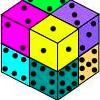### Six Numbered Cubes

##### Age 7 to 11 Challenge Level:

This task combines spatial awareness with addition and multiplication.### More Magic Potting Sheds

##### Age 11 to 14 Challenge Level:

The number of plants in Mr McGregor's magic potting shed increases overnight. He'd like to put the same number of plants in each of his gardens, planting one garden each day. How can he do it?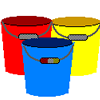### Buckets of Thinking

##### Age 7 to 11 Challenge Level:

There are three buckets each of which holds a maximum of 5 litres. Use the clues to work out how much liquid there is in each bucket.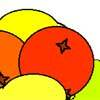### Oranges and Lemons

##### Age 7 to 11 Challenge Level:

On the table there is a pile of oranges and lemons that weighs exactly one kilogram. Using the information, can you work out how many lemons there are?### Calendar Calculations

##### Age 7 to 11 Challenge Level:

Try adding together the dates of all the days in one week. Now multiply the first date by 7 and add 21. Can you explain what happens?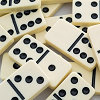### Amy's Dominoes

##### Age 7 to 11 Challenge Level:

Amy has a box containing domino pieces but she does not think it is a complete set. She has 24 dominoes in her box and there are 125 spots on them altogether. Which of her domino pieces are missing?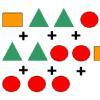### Super Shapes

##### Age 7 to 11 Short Challenge Level:

The value of the circle changes in each of the following problems. Can you discover its value in each problem?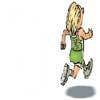### Rocco's Race

##### Age 7 to 11 Short Challenge Level:

Rocco ran in a 200 m race for his class. Use the information to find out how many runners there were in the race and what Rocco's finishing position was.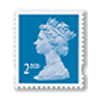### Penta Post

##### Age 7 to 11 Challenge Level:

Here are the prices for 1st and 2nd class mail within the UK. You have an unlimited number of each of these stamps. Which stamps would you need to post a parcel weighing 825g?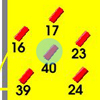### Throw a 100

##### Age 7 to 11 Challenge Level:

Can you score 100 by throwing rings on this board? Is there more than way to do it?### Bundles of Cubes

##### Age 7 to 11 Challenge Level:

Watch this animation. What do you notice? What happens when you try more or fewer cubes in a bundle?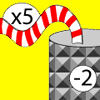### Function Machines

##### Age 7 to 11 Challenge Level:

If the numbers 5, 7 and 4 go into this function machine, what numbers will come out?### Being Resilient - Primary Number

##### Age 5 to 11 Challenge Level:

Number problems at primary level that may require resilience.### Method in Multiplying Madness?

##### Age 7 to 14 Challenge Level:

Watch our videos of multiplication methods that you may not have met before. Can you make sense of them?### The Deca Tree

##### Age 7 to 11 Challenge Level:

Find out what a Deca Tree is and then work out how many leaves there will be after the woodcutter has cut off a trunk, a branch, a twig and a leaf.### Sending Cards

##### Age 7 to 11 Challenge Level:

This challenge asks you to investigate the total number of cards that would be sent if four children send one to all three others. How many would be sent if there were five children? Six?### Clock Face

##### Age 7 to 11 Challenge Level:

Where can you draw a line on a clock face so that the numbers on both sides have the same total?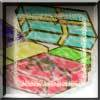### Gift Stacks

##### Age 7 to 11 Challenge Level:

Use the information to work out how many gifts there are in each pile.### Number Tracks

##### Age 7 to 11 Challenge Level:

Ben’s class were cutting up number tracks. First they cut them into twos and added up the numbers on each piece. What patterns could they see?### Tom's Number

##### Age 7 to 11 Challenge Level:

Work out Tom's number from the answers he gives his friend. He will only answer 'yes' or 'no'.### Oh! Hidden Inside?

##### Age 11 to 14 Challenge Level:

Find the number which has 8 divisors, such that the product of the divisors is 331776.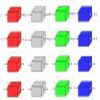### Machines

##### Age 7 to 11 Challenge Level:

What is happening at each box in these machines?### Repeaters

##### Age 11 to 14 Challenge Level:

Choose any 3 digits and make a 6 digit number by repeating the 3 digits in the same order (e.g. 594594). Explain why whatever digits you choose the number will always be divisible by 7, 11 and 13.### Looking at Lego

##### Age 7 to 11 Challenge Level:

This task offers an opportunity to explore all sorts of number relationships, but particularly multiplication.### Difficulties with Division

##### Age 5 to 11

This article for teachers looks at how teachers can use problems from the NRICH site to help them teach division.### Power Mad!

##### Age 11 to 14 Challenge Level:

Powers of numbers behave in surprising ways. Take a look at some of these and try to explain why they are true.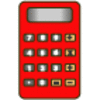### Clever Keys

##### Age 7 to 11 Short Challenge Level:

On a calculator, make 15 by using only the 2 key and any of the four operations keys. How many ways can you find to do it?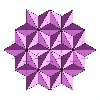### Next Number

##### Age 7 to 11 Short Challenge Level:

Find the next number in this pattern: 3, 7, 19, 55 ...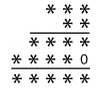### Long Multiplication

##### Age 11 to 14 Challenge Level:

A 3 digit number is multiplied by a 2 digit number and the calculation is written out as shown with a digit in place of each of the *'s. Complete the whole multiplication sum.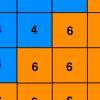### Six Ten Total

##### Age 7 to 11 Challenge Level:

This challenge combines addition, multiplication, perseverance and even proof.### Surprising Split

##### Age 7 to 11 Challenge Level:

Does this 'trick' for calculating multiples of 11 always work? Why or why not?### What Is Ziffle?

##### Age 7 to 11 Challenge Level:

Can you work out what a ziffle is on the planet Zargon?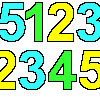### 1, 2, 3, 4, 5

##### Age 7 to 11 Challenge Level:

Using the numbers 1, 2, 3, 4 and 5 once and only once, and the operations x and ÷ once and only once, what is the smallest whole number you can make?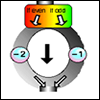### The Number Crunching Machine

##### Age 7 to 11 Challenge Level:

Put a number at the top of the machine and collect a number at the bottom. What do you get? Which numbers get back to themselves?### A One in Seven Chance

##### Age 11 to 14 Challenge Level:

What is the remainder when 2^{164}is divided by 7?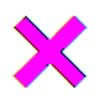### Learning Times Tables

##### Age 5 to 11 Challenge Level:

In November, Liz was interviewed for an article on a parents' website about learning times tables. Read the article here.### Square Subtraction

##### Age 7 to 11 Challenge Level:

Look at what happens when you take a number, square it and subtract your answer. What kind of number do you get? Can you prove it?### Remainders

##### Age 7 to 14 Challenge Level:

I'm thinking of a number. My number is both a multiple of 5 and a multiple of 6. What could my number be?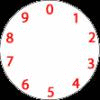### Dividing a Cake

##### Age 7 to 11 Challenge Level:

Annie cut this numbered cake into 3 pieces with 3 cuts so that the numbers on each piece added to the same total. Where were the cuts and what fraction of the whole cake was each piece?